# Discuss the shape of the following molecules using VSEPR model

Discuss the shape of the following molecules using VSEPR model.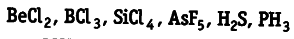According to VSEPR theory, the shape of a molecule depends upon the number of valence shell electron pairs (bonded or non-bonded) around the central atom. Pairs of electrons in the valence shell repel each other. The order of their repulsions is as follows
Ip — lp> Ip — bp > bp — bp
(i) { BeCl }_{ 2 }
The central atom Be has only 2 valence electrons which are bonded to Cl, so there are only 2 bond pairs and no lone pairs. It is of the type { AB }_{ 2 } and hence, the shape is linear.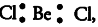(ii) { Bcl }_{ 3 }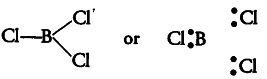The central atom B has only 3 valence electrons which are bonded with three Cl atoms, so it contains only 3 bond pairs and no lone pair. It is of the type { AB }_{ 3 } and hence, the shape is trigonal planar.
(iii){ SiCl }_{ 4 }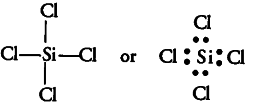Similarly, the central atom Si has only 4 bond pairs and no lone pair. It is of the type { AB }_{ 4 } and hence, the shape is tetrahedral.
(iv){ AsF }_{ 5 }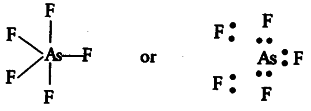The central atom As has only 5 bond pairs and no lone pair. It is of the type { AB }_{ 5 } and hence, the shape is trigonal bipyramidal.
(v)H _{ 2 }S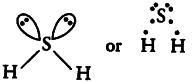The central atom S has 6 valence electrons. Out of these only two are used in bond formation with two H-atoms while four (two pairs) remains as non-bonding electrons (i.e., lone pairs). So, it contains 2 bond pairs and 2 lone pairs. It is of the type AB_{2}E_{2} and hence, the shape is bent or V- shaped.
(vi){ PH }_{ 3 }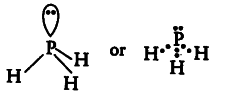The central atom P has 5 valence electrons. Out of which three are utilised in bonding with H atoms and one pair remains as lone pair. So, it contains 3 bond pairs and one lone pair. It is of the type { AB }_{ 3 }E and hence the shape is pyramidal.